# SAT Math Multiple Choice Question 346: Answer and Explanation

### Test Information

Question: 346

1.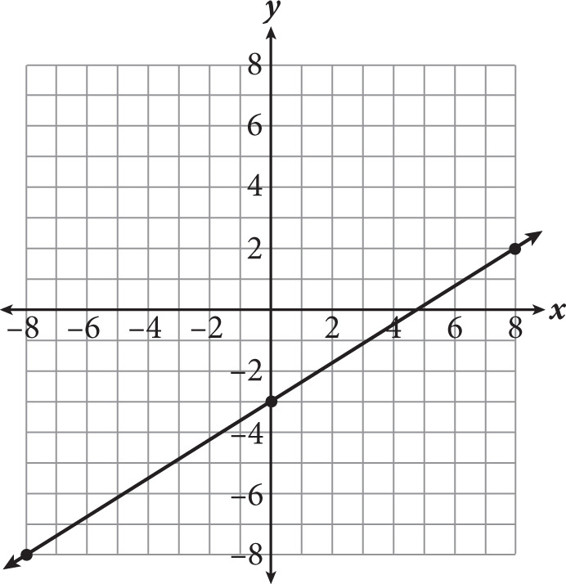What is the average rate of change for the line graphed in the figure above?

• A.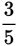• B.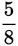• C.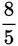• D.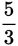Getting to the Answer: Find the slope of the line by either using the slope formula or by counting the rise and the run from one point to the next. If you start at (0, -3), the line rises 5 units and runs 8 units to get to (8, 2), so the slope, or average rate of change, is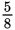.